## Table of ContentOpen Access

ARTICLE

# Energy-Efficient Routing Protocol with Multi-Hop Fuzzy Logic for Wireless Networks

J. Gobinath1,*, S. Hemajothi2, J. S. Leena Jasmine3

1 Department of Information and Communication Engineering, Anna University, Chennai, 600025, India
2 Department of Electronics and Communication Engineering, Vel Tech High Tech Dr. Rangarajan Dr. Sakunthala Engineering College, Chennai, 600062, India
3 Department of Computer Science and Engineering, Velammal Engineering College, Chennai, 600066, India

* Corresponding Author: J. Gobinath. Email:Intelligent Automation & Soft Computing 2023, 36(2), 2457-2471. https://doi.org/10.32604/iasc.2023.031171

## Abstract

A Wireless Sensor Network (WSN) becomes a newer type of real-time embedded device that can be utilized for a wide range of applications that make regular networking which appears impracticable. Concerning the energy production of the nodes, WSN has major issues that may influence the stability of the system. As a result, constructing WSN requires devising protocols and standards that make the most use of constrained capacity, especially the energy resources. WSN faces some issues with increased power utilization and an on going development due to the uneven energy usage between the nodes. Clustering has proven to be a more effective strategy in this series. In the proposed work, a hybrid method is used for reducing the energy consumption among CHs. A Fuzzy Logic-based clustering protocol FLUC (unequally clustered) and Fuzzy Clustering with Energy-Efficient Routing Protocol (FCERP) are used. A Fuzzy Clustering with Energy Efficient Routing Protocol (FCERP) reduces the WSN power usage and increases the lifespan of the network. FCERP has created a novel cluster-based fuzzy routing mechanism that uses a limit value to combine the clustering and multi-hop routing capabilities. The technique creates uneven groups by using fuzzy logic with a competitive range to choose the Cluster Head (CH). The input variables include the distance of the nodes from the ground station, concentrations, and remaining energy. The proposed FLUC-FCERP reduces the power usage and improves the lifetime of the network compared with the existing algorithms.

## Keywords

1  Introduction

Wireless Sensor Networks (WSNs) have attracted attention due to their wide use of applications ranging from disaster response, healthcare, and home appliances. In particular, miniaturized wireless sensors were made possible by advances in micro-electrical-mechanical, wireless technology, and computer technologies. WSN is made up of several small sensing devices. Such devices are placed to watch certain potential targets in a deterministic/random manner. The sensor nodes expend a great deal of energy when detecting, analyzing, and transferring the gathered information. Those nodes come with a lower voltage that is both distinctive and reusable .

Since the network nodes contain minimum energy, a considerable amount of energy is used in WSN while transmitting the data. As a result, a design that uses minimal energy when delivering the information to Bs must be considered. One of the ways to save energy is to use a hierarchical structure in system architecture. The system components in a hierarchical structure are grouped into numerous levels, with the nodes for each level possessing the same features. Clustering is one of the approaches to constructing a hierarchical system. Therefore, the network elements are subdivided into different clusters assuring all sensor nodes collect information from their immediate surroundings .

The power of networks should be used carefully for the life of the channel. Among the most effective approaches to reduce network power consumption is to organize sensors network into clusters based on a common feature. A Cluster Head (CH) is located at the center of each cluster and permits the connection with such an access point (BS). The CHS collect information from the other components in their clusters . Multi-hop or common communication modes are used in CH-BS communications. A CH interacts directly with BS in the latter form. Multi-hop transmission, on either side, is defined as CH transferring the data across the other CHs to finally reach the BS .

In addition, the clusters in the multi-hop communications minimize a lot of connections, minimizing latency. Furthermore, multi-hop communication enables the cluster members to support CH in information fusion, hence reducing the power consumption of CH. It will contribute to the life span of the system . On the other hand, the CHs that are the closest to BS, are characterized by significant information processing in multi-hop communications. It is referred to as a “hot zone” of power . The unequal clustering methods can be used to prevent power gap issues. The strategies involve making the clusters near the BS minimal in size compared to clusters further off. This guarantees that CHs (close to the BS) are better prepared to distribute the internet traffic.

The majority of WSN grouping research has concentrated on developing centralized and decentralized procedures in compute clusters of CHs. In complex systems, though, centralized systems are ineffective since gathering all of the important details just at the main BS is power and time taking . In large-scale networks, distributed protocols are even more effective. Nodes might choose to imitate a CH or a change in the existing clusters. This type of data that blocks from the nearby nodes determines the procedure. In the research, many scattered clustering protocols are proposed. The probabilistic evaluation of CH selection characterizes the probability procedures. Recursive methods use an iterative algorithm on the networks to determine whether or not a CH should be created. Clustering methods can indeed be dynamic or static, depending on their nature. In a stable grouping, the groups are persistent. The method is divided into separate stages in dynamic clustering. For the next round, the clusters are created again. Whenever a recurrent cluster is generated on the network, there is an extra cost .

Fuzzy logic is used extensively in several protocols . In a fuzzy inference system, real-time judgments can be made even when the external conditions are unknown. Another major application of fuzzy logic is the combination of multiple environmental characteristics based on predefined criteria and subsequently arriving at a judgment based on the results. Fuzzy clustering algorithms, on the other hand, apply the same reasoning and can choose CHs by integrating two grouping systems. Fuzzy logic is just a framework that works in a manner that is almost identical to rational reasoning. The fuzzifier, defuzzifier, fuzzy rules, and inferences processors are the four main components of fuzzy logic .

The main contributions of the proposed FLUC-FCERP method are given below:

•   This method provides a decentralized fuzzy logic technique to compute the cluster radius of unequally sized clusters.

•   Wireless Sensor Networks employ multi-hop transmissions with uneven grouping to reduce power usage.

•   The proposed Fuzzy Based Clustering and Energy-Efficient Routing Protocol (FCERP) is to provide a simple and easy power routing algorithm based on clustering with a fixed threshold.

•   FCERP implements multi-hop and the selection of the best intermediate nodes.

•   The intermediary point is selected among the listed headers network based on the competence criterion of “distance to BS” and “leftover network power.”

The rest of the paper is organized in sections as shown. Section 2 consists of a brief study of existing Wireless Sensor Networks, Energy consumption, and Fuzzy Logic. Section 3 describes the working principle of the proposed model. Section 4 evaluates the result and gives a comparison of different algorithms. Section 5 concludes the research work with the future scope.

2  Literature Survey

The Multi-Objective Fuzzy Clustering Technique (MOFCA)  is a fuzzy clustering method that generates fuzzy variables such as “remaining energy,” “length to BS,” and “intensity of every node.” This technique uses a competing energy-based perimeter to select the CH. The purpose of this method would have been to overcome the problems of the “power gap” and “warm area.” Utilizing Half Nodes Alive (HNA), First Node Dead (FND), and Overall Remain Power, this method computes the lifetime of WSNs (TRE). The leftover power from the terminals is used to calculate the CHS radius depending on its position from the BS. To handle the situation of WSN utilizing efficient methods, the concentration of parameters is defined as input variables for the constructed fuzzy logic.

In WSNs, low energy adaptive clustering hierarchy (LEACH) is a clustering technique in which the sensor networks are based on probabilities that are chosen as feasible CH. Every node chooses a random value. If the specified value is less than the defined threshold, a node becomes the CH immediately. Non-CH networks joined the CHs after the CHs have been identified based on the distance. The efficiency of an ambitious CH is originally poor. Furthermore, the remaining energy is not taken into account while choosing a CH. This is incompatible with the effective operation of a heterogeneity network. The use of the location of data from nodes to pick the CH has several drawbacks. Furthermore, this technique includes low-energy sites which may be selected as CHs, causing their power to be depleted even faster.

PEGASIS is a string, located to the close system which is an improvement to LEACH. Every node connects with a nearby node in this method. The terminals switch off transmitting the information to the BS. The energy consumption of each round is reduced as a result of this procedure. One of the primary flaws of the system is that the CHs communicate the information to the Bs immediately. It is a single network technique that uses a lot of energy and it is unsuitable for a large-scale system like WSN.

The energy-aware and multi-hop intracluster hierarchical (EAMMH) standard protocol was created by combining multi-hop intra-clustering and energy-aware navigation characteristics . The use of the EAMMH procedure was broken down into stages. Every round began with a releasing stage in which the clusters were organized. The stable stage, which involves data transmission to the base station BS, followed the first process. The sensor nodes were organized into groups in the second stage, which resulted in multi-hop intra-cluster communications. From each node to the CH, many pathways were constructed. It allowed for an energy-aware heuristics connection, in which the best route was selected. As a result, the proposed method selected the CHs based on their remaining energy, particularly when the life duration of the program is limited.

Durable routing flexible study automaton (RRDLA) method was developed in , which selects the minimum number of sensor nodes to maintain the given QoS parameters. Different quality of services (QoS) routing limitations, including edge dependability and latency, were factored during the router selection. These writers  used an Irregularity Cellular Learning Automaton (ICLA)-based method, which is also known as SPLA (automation tool), to defend the security of the sensors and provide WSN performance. The goal of the training machine at every irregular cell learning automata (ICLA) cell with suitable rules is to study the smallest number of sensor nodes to secure the self-protection criteria of the 0 channel.

The energy-aware fuzzy clustering (EAFCA) method [18,19] suggested the utility of the fuzzy method to better cluster in a WSN. Multi-hop communication is being used for transmitting the meaning to the CH in every cluster, as well as the competitive range of every group is two jumps. The CH is determined by the “remaining energy,” “concentration of every node,” and “centric frequency” characteristics. According to scientists, in a sensing system, network nodes are partial or full community nodes. A powerful method for CH data and information gathering could be designed. Lastly, the study concluded the Spatio temporal connections  in the information created by sensor networks might be tweaked. Improving the effectiveness of this system is done by recommending a sleep/wake-up plan for the networks depending on the evidence produced by them.

The researchers suggested the increase of data gathering effectiveness in two detection systems in Two-Tier Distributed Fuzzy Logic-Based Protocol (TTDFP) . Initially, the best CH was chosen using the probability models. Instead of using a trial-and-error technique to determine the optimal balance of these variables, TTDFP is used. The TTDFP uses the optimization model to tweak the two values in this layer, the criterion diameter, and the maximal competitiveness diameter. For group size calculation, fuzzy-based unequal clustering (FBUC) [22,23] uses three variables; residual energy, proximity to BS, and node density, This method leverages fuzzy logic by allowing the non-cluster sites to connect with CH. The CH competitive areas and the gap between CH and non-cluster individuals were used in this approach to choosing CHs.

An Energy-Efficient Fuzzy Logic Cluster Head (EEFL-CH) method was developed as an upgrade to the Leach algorithm . The goal of this approach was to use fuzzy logic to minimize power usage while extending the lifetime of the system. These different fuzzy factors of remaining energy predicted the effectiveness and the proximity to an access point that is used to choose the CH. The researcher LEE  suggested a fuzzy-logic-based clustering method with just an application to energy prediction that improves the lifetime of the network by spreading the workloads equitably. With selected CHs, this method employs two fuzzy variables; residual power and predicted remaining power.

3  Proposed Fuzzy Unequal Clustering Methodology

In the proposed work Fuzzy Logic-based clustering protocol FLUC (unequally clustered) and Fuzzy Clustering with Energy-efficient routing protocol (FCERP) method were used. It consisted of the System model, Network Model, and Energy Model. Fig. 1 shows the architecture of the proposed system. The first data was collected from wireless nodes. Then the unequal clustering was done by the Fuzzy algorithm. Finally, the clusters were formed, and the proposed FCERP was used to transfer the data with less power consumption.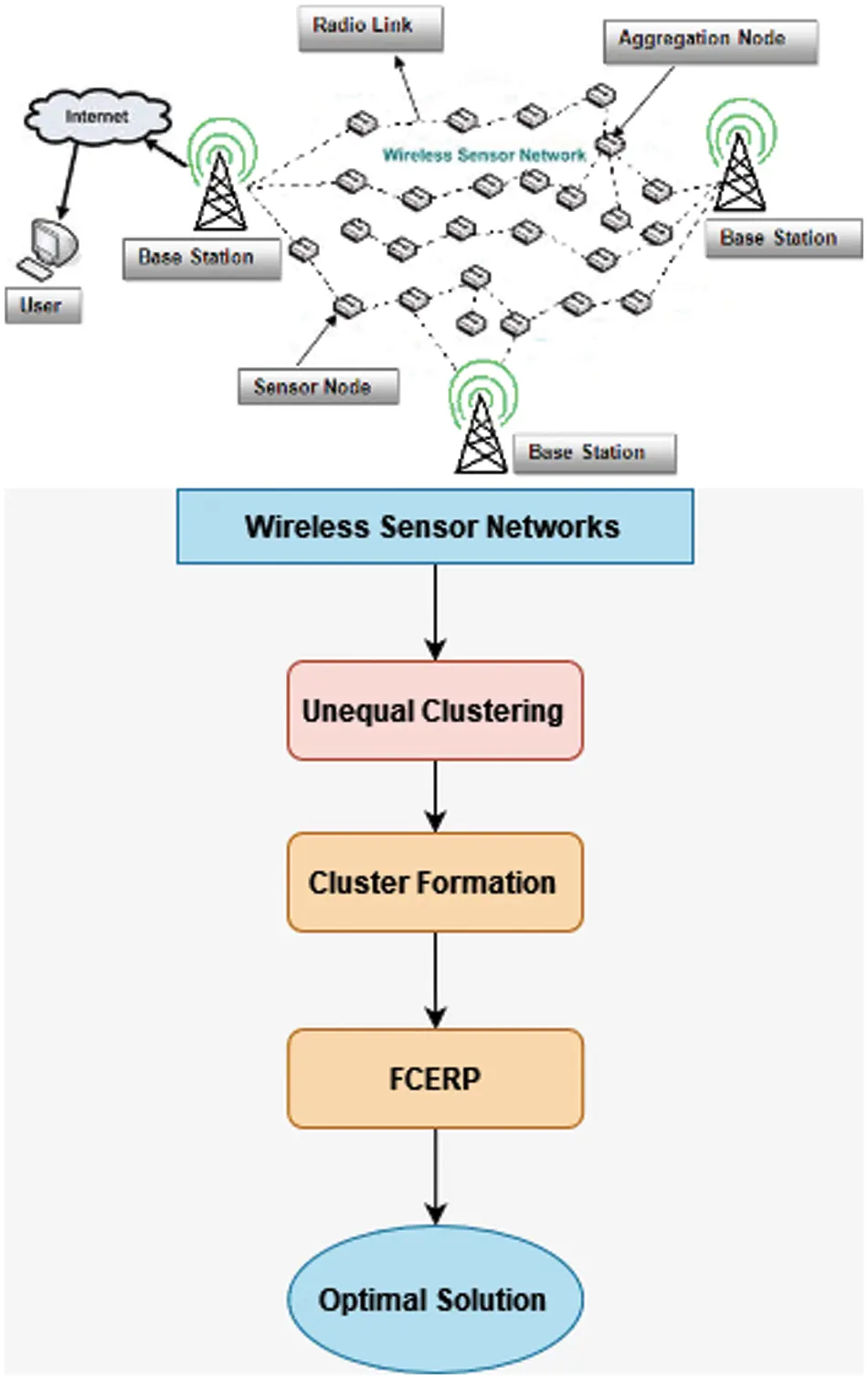Figure 1: Architecture of the proposed system

3.1 System Model

Below were the characteristics that supported the suggested FCERP:

•   Every node had the same beginning power, and its content was homogeneous.

•   The placement of the nodes in the network was chosen at randomness.

•   The network nodes were thought at the same moment.

•   Both vertices and BSs were in a stationary condition.

•   Euclid’s method was used to calculate the length.

•   BS got the display in many jumps and as a single connection under specific circumstances.

•   The neighbors of a cluster were vertices that were put at a range of R from such a point.

The transmission power estimation for sending “L”-bit packets of data from the sender to the receiver at a range of “D” between them was as follows:

Entx(L,D){LEnelec+LϵfsD2ifD<D0LEnelec+LϵmpD4ifD>D0 (1)

The following formula is used to determine the value of D0 :

D0=εfsεmp (2)

Here Enelec is the quantity of electricity spent by the transmitter while transmitting every part of information communicated to the receiver. The parameter fs is used to calculate the power usage for the outside transmissions, whereas the parameter is used to calculate the power usage for multi-hop communication. This sign, which would be derived using given equations, provides enough energy needed by the receivers to collect the messages.

EnRX=LEnelec (3)

3.2 Network Model

The networks are evaluated in which a quantity of homogenous edge devices is arbitrarily distributed in a region and BS is situated even outside the entire network. Appropriate assumptions are followed in order to develop the suggested network approach:

•   Following the placement, all sensors and the ground station are deemed fixed.

•   Sensor networks can only connect to a particular CH within their transmitting distance.

•   BS is not limited to power.

•   Wireless network is continuous and symmetrical.

•   At first, every sensor network has the same total energy.

3.3 Energy Model

Assume that the nodes in the M * M area are scattered evenly. Whether there are k clusters, the mean number of nodes in each cluster is N/K. Every cluster occupies about M2/K of the available space. The necessary square length between the nodes and the CH is calculated as follows:

En[DtoCH2]=ρ(X,Y)DXDY (4)

Now consider that a region is a circular with radius R=(MπK) and that ρ(r,θ) is constant for r and θ , thus the Eq. (1) becomes

En[DtoCH2]=ρθ=02πr=0MπKr3rθ=ρ2πM4K2 (5)

The size D=2MπK and the frequency of the networks are equal across the clustered region if the population of the node is equal all through the clustered region.

En[DtoCH2]=12πM2K (6)

The range among CH nodes and BS on median

DtoBS=A1X2+Y21AA=0.765M2 (7)

DtoBS=0.755M2 (8)

To begin, it is assumed that all the clusters have the same size, thus the diameter of every cluster is R, and the area of each cluster is M/K, so the diameter of the clusters is R=MπK . The dimension of the cluster, or the distance of the two cluster heads, has become D=2MπK .

The signal-to-noise ratio (SNR) whilst transmitting a l-bit payload across a range of d is calculated as follows:

EnTX(L,D)={ LEnelec+LεfsD2,    ifDD0LEnelec+LampD4,     ifDD0(9)

when Enelec is for power drained of each bit to function the electronics, fs and amp stand for space available and multi-fading channels power usage, D0 stands for range between the transmitter and the recipient, and D0 stands for the threshold data transmission. Trying to equate the formula for D=D0 that yields the threshold distance D0.

LEnelec+LfsD02=LEnelec+LampD04 (10)

3.4 Unequal Clustering

In order to save the power in the wireless connection, sensor networks choose CH forwarding their information. Because one of the key criterion in the selection of node as CH is its proximity to BS, a great amount of CM nodes which could choose it for message transmission, causing CH’s batteries to deplete quickly. Even the range of node from BS grows, the amount of energy needed for data transfer grows as well, resulting in rapid battery loss. An uneven clustering method was suggested in which the radius of the CH fluctuates the solution and the farthest distance to BS for preventing quick charge depletion of CH. Throughout this technique, candidates CHs were chosen first, and then ultimate CHs for transmitting the data depending on other characteristics. The following is a description of the system. Basic CH and temporary CH were selected in this method using a prediction method. For each grouping cycle, an arbitrary figure was generated by each sensing node between the two discrete integers (0 and 1) for the first CH selection. If a node’s random numbered will be less than the threshold (T), that particular node will be the first CH on its own. In the suggested paradigm, the competition radius from each provisional CH varies constantly.

The developed model calculates its wide range of radius using node density, proximity to the BS, and remaining energy. As a result, it makes sense to lower the network region for CH and its remaining energy is dropped correspondingly. If the competitive circle doesn’t really decrease whereas the leftover energy of sensor node decreases, the sensor network will quickly lose its power. To manage the uncertainty, the preset fuzzy IF-THEN outlined regulations are used to calculate the diameter.

Initially, CHs are chosen at each round by allocating a set of numbers to each node. If a Threshold Value (TH) of nearby nodes assigned in Eq. (12) is smaller than the created random number assignment, the cluster has become a temporary CH.

TH=P/(1P(rmod1/p)) (11)

Here r denotes the current round and the value and P denotes the preferred proportion of CH (e.g., P = 0.05). This chapter will be using fuzzy logic to explain the unequal clustering process. Unlike all the other researches, the proposed model had employed three fuzzy linguistics. The power rating in the preliminary CH, proximity to a BS, and intensity were the explanatory variables considered.

The part contained an explanation of the fuzzy logic-based unequal clustering procedure. Three factors were employed in the current study. The variables used were the provisional CHs’ residual energy, distances to the BS, as well as the density of nodes near to the CH. There was only one output variable in the previous fuzzy-based uneven clustering procedure. Two output parameters were included in the proposed method. CH likelihood and its competitive area were all these. With the complex exponential values, the variables were extremely huge and exceedingly tiny. The remaining tasks were triangle in nature.

The first factor was composed of seven linguistic variables that were all hazy. Using triangular membership equations, the answers were both effective and ineffective. The remaining was characterized by triangular functions. Every node was meant to be subjected to a repeating experiment conducted on different networking magnitudes due to the cautious choice of membership value extent. A fuzzy theory algorithm fuzzifies the precise data points into linguistic terms that are more appropriate.

μA1(X)={0Xa1Xa1b1a1a1Xb1c1Xc1b1b1Xc10c1X (12)

μA2(X)={0Xa2Xa2b2a2a2Xb21b2xc2d2Xd2c2c2Xd20d2x (13)

3.5 Formation of Cluster

The sensor network generates a random point among 1 and 0 there at start of each round. When random number of the node is much less than the Thresholds (TH) value, node is designated as a temporary CH. That applies to a wide range radius which is calculated using the node’s length from the BS, remaining energy, and intensity. Hotspot problems may arise in some circumstances when the number of groups and inter-cluster connectivity grow. The number of nodes with two tiny diameters may be created as a result of this issue. As a remedy to this problem, the radius was reduced to avoid the system from being too fragmented.

After that, the preliminary CH would calculate the diameter and the probability. Each node inside the cluster diameter sends out CH-MSG to create its forwarding table, which shows a collection of neighbors and remaining energy. The CH will send CH-MSG to the neighbors within a radius set by FIS. The probability number of the node and id will be included in this tentative CH-MSG. The Prime cluster head is the Provisional CH with the highest chances number within the cluster (PCH). ELECTED-CH-MSG is subsequently relayed to the nearby nodes by PCH. JOIN-CH-MSG will be relayed to the nearby CH by the total number of nodes which do not form PCH. Lastly, the PCH sends a message towards its cluster members with a transmission time tables. The cluster members deliver raw information to the PCH by these slots’ tables. Its package transfer is identical to EAMMH and EAUCF methods after grouping is completed. As a result, this paper does not go into great detail about the sending data and synchronization processes in the cluster.

Because it uses the power, a smaller range towards the BS, and decreased level in the variables for CH probability computation, there commended temporary CH competition perimeter of the system changes dynamically. Lowering the CH range is the most efficient way to save money. The CH chance is determined by the configured fuzzy IF-THEN rules. Unpredictable nature is included into the WSN and effectively computed utilizing the fuzzy method. The fuzzy numbers which are similar are stored in the input parameter. Closer, distant, most far as fuzzy linguistic variables characterize the position of the node to the BS in the original input parameter. These values of the farthest and the closest are trapezoid; whereas the values of differences are statistically significant and closest are triangles. The second input parameter, nodes based on residual, has the same attributes as the linguistic terms; lower, medium, and higher. The roles of high and low are trapezoidal. The similarity measure of the mean is triangle. Its final input parameter is intensity that has the characteristics of minimal, moderate, and large. The roles of low and high are trapezoidal.

3.6 Fuzzy System

Whenever it relates to grouping, choosing a CH node is among the most major decisions to be accounted. By identifying the most suitable nodes to operate as CH, a great deal of energy is saved and extended the life of the system. Many methods for choosing the CH node have already been suggested up involving the probability choosing, definitive classification, evolutionary computation, and use of fuzzy inference system in the recruitment process. Fuzzy systems minimize the complexities associated with WSNs.

Fuzzy set theory is a multi-value reasoning whereby the proper value of each statement might range from zero to one. A fuzzy process utilizes the fuzzy logic to methodically convert a base knowledge into a non-linear mapping. The primary design of the fuzzy inference system is continued as follows and its functioning is shown in Fig. 2.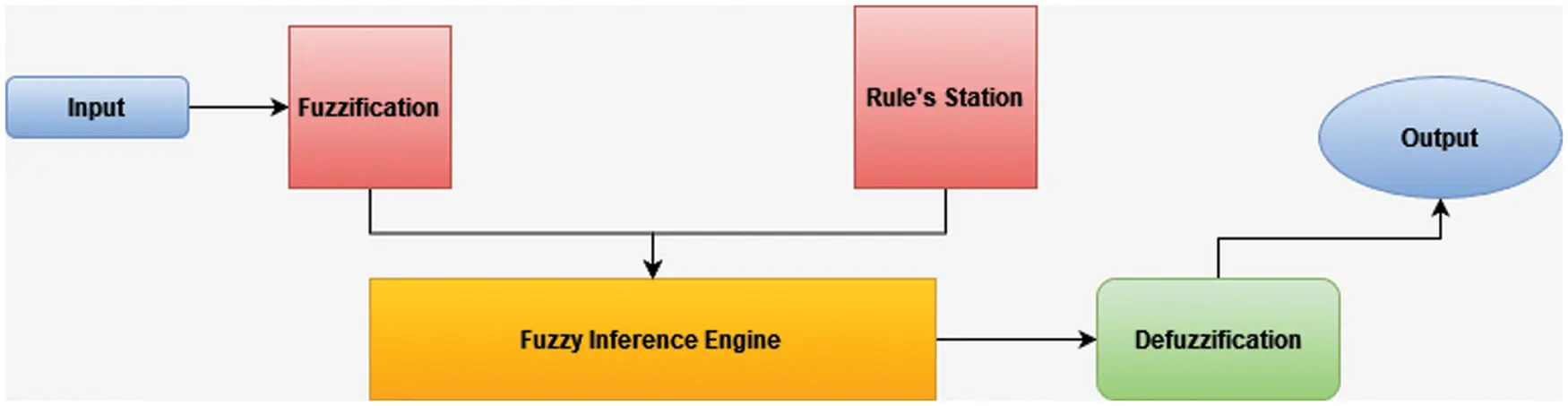Figure 2: Design of fuzzy system

3.7 Fuzzy Clustering and Energy Efficient Routing Protocol

Clustering and energy-efficient routing using fuzzy system are the two components of the FCERP. It reduces the clusters in WSN using a predetermined limit, a range of grouping, and a combination technique for transmitting the data to Bs for improving the performance of WSN. The suggested FCERP has the following basic features.

•   For each cycle, fuzzy scheme distributing grouping, uneven clusters, and no clustering are used to minimize the overall energy usage and the quantity of control packets broadcast.

•   Every cluster has its own collection of fuzzification variables for determining an optimal material component on the remaining energy and its specific address inside the clusters.

•   Setting a preset threshold for their power level has been studied as a way to reduce the number of header routers re-clustering.

•   The method determines the best path for transmitting the messages out of each headers node to the BS using a multi-hop method.

The reduced power consumption within the sensor nodes is among the most essential factors to consider when compared to the clustering techniques. Lowering the number of powers consumed can help the system to run more efficiently. As a result, as grouping density increases even as the number of nearby nodes grows, each node of the neighbor’s number is treated as the secondary fuzzy variable of the cluster. This power consumption of the program remains steady whenever the node of the cluster has a uniform dispersion. As a result, the remaining energy of each node and the quantity of the neighbor have considered the fuzzy input variables for the first clusters, which would be treated in the cycles of 1, 4, and 7, until the last group is constructed and handled. Fig. 3 shows the architecture of FCERP protocol.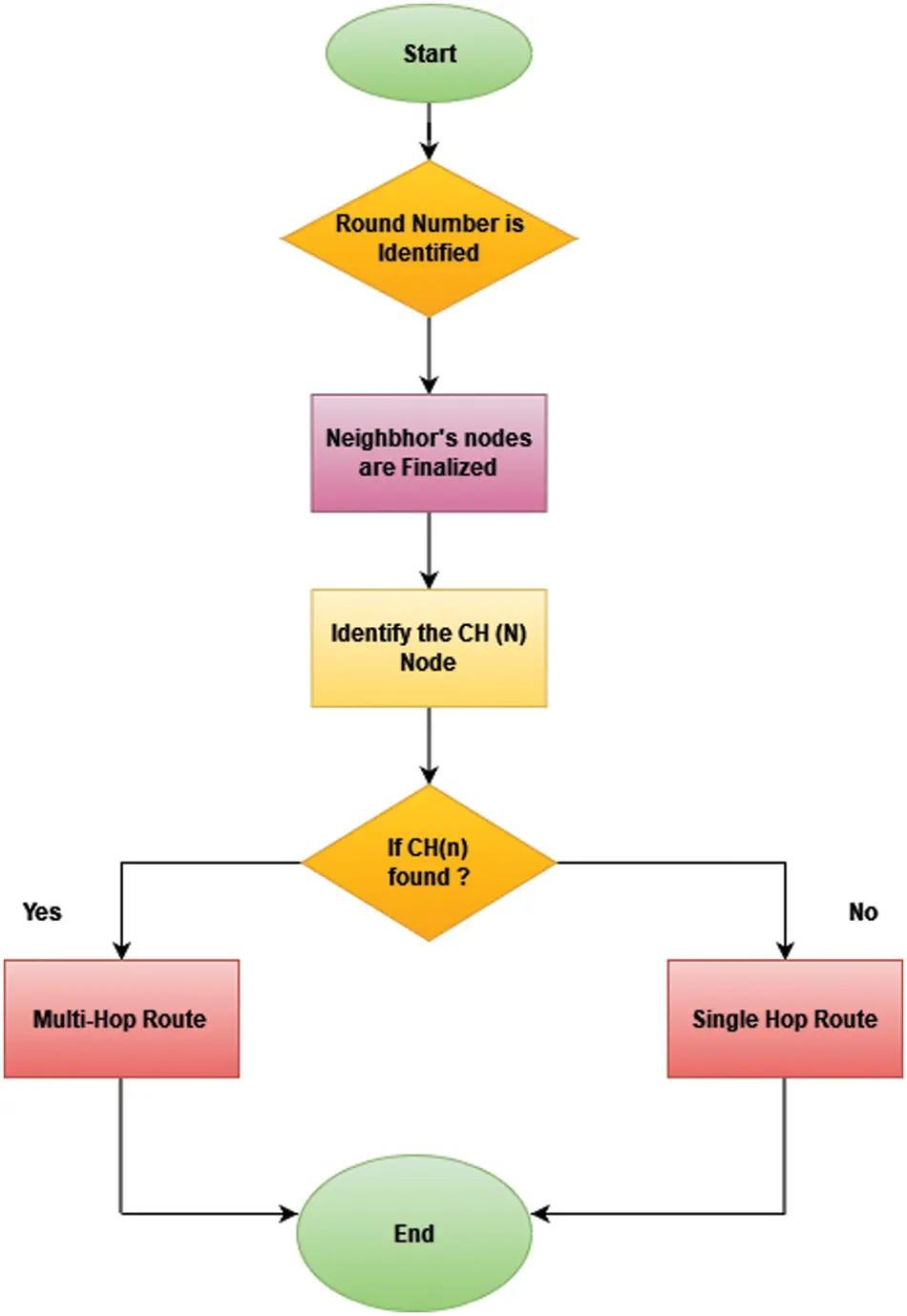Figure 3: Architecture of FCERP

It is to save the power that the networking protocols that are proposed are being developed. By implementing an efficient scheduling plan, the proper number of nodes distance are calculated among the nodes as well as the BS that assist the way of enhancement and networks longevity. For transmitting the information to BS, this article uses a multi-hop approach. The nodes have collected the received data in the suggested FCERP by performing grouping in each round and delivering the sensed information in the Network. It transfers it to the BS through the CH node in the multi-hop mode. The CH node is picked among the identified CHs depending on the concepts in order. The network node “range to BS (Di)” and “remaining energy (RE)” are combined to form the Competence Measure (CM).

CM(CH(i))=Di(CH(i))RE(CH(i)) (14)

A competitive radius (Rc) should be computed to choose a head node between the present CHs.

Rc=Di(CH(i))2 (15)

4  Result Analysis

The suggested approach was tested in MATLAB, which provided a Fuzzy Toolbox that had checked all the fuzzy membership functions, making it appropriate for applications. MATLAB was used to evaluate the proposed technique. After being distributed over a 100 100 m2 area, 100 sensor nodes were examined. The starting energy in each node was assumed to be 0.5 J. Table 1 lists the MATLAB simulation frameworks that were used in the proposed system.The methods were assessed using the lifetime of the network measure, which includes the variables of FND, HND, and LND, and the amount of energy usage in each round and also the amount of dead node in each round. The outcomes of the evaluating methods known as the network lifespan are shown in Figs. 46. Considering the aspects of the First Node Dies (FND), Half Node Dies (HND), and Last Node Dies (LND), the suggested FLUC-FCERP surpasses the previous techniques. The algorithms such as LEACH, EAUCF, FCERP and FLUC-FCERP were compared.Figure 4: Lifetime of the network using FND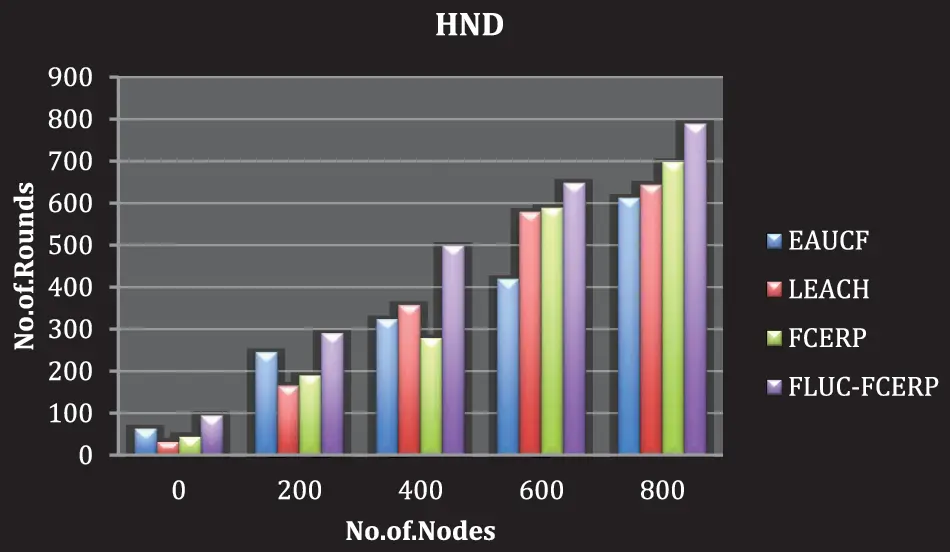Figure 5: Lifetime of the network using HND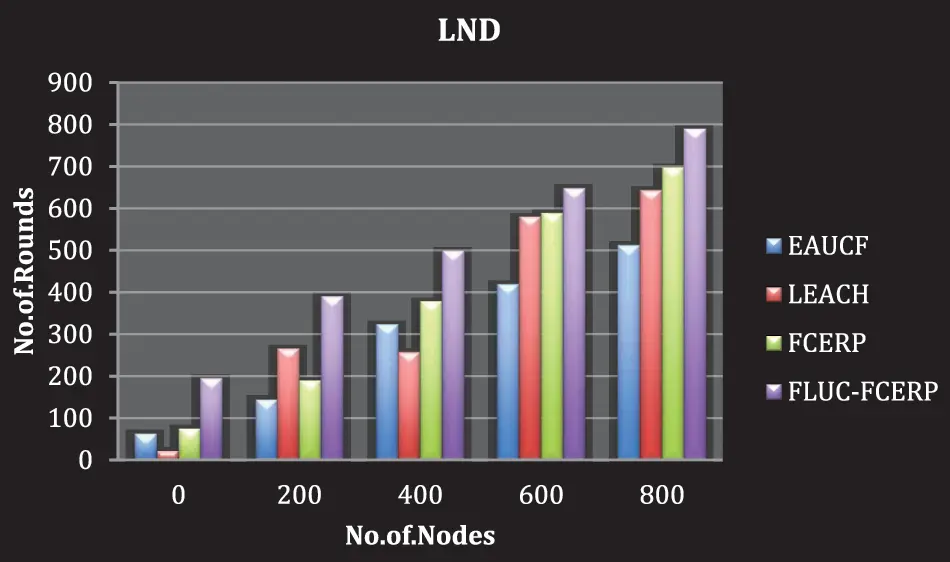Figure 6: Lifetime of the network using LND

In all the three parameters (LND, FND and HND), the proposed method achieves better lifetime of the network. As shown in Fig. 7, transferring the multi-hop data out of each CH to the BS and lower than the total of clustering occurrences seems to reduce the amount of control messages transmitted while ensuring a balancing power usage and decreases the number of dead nodes in each cycle. Moreover, the efficiency of the suggested method is much more constant than the traditional algorithms.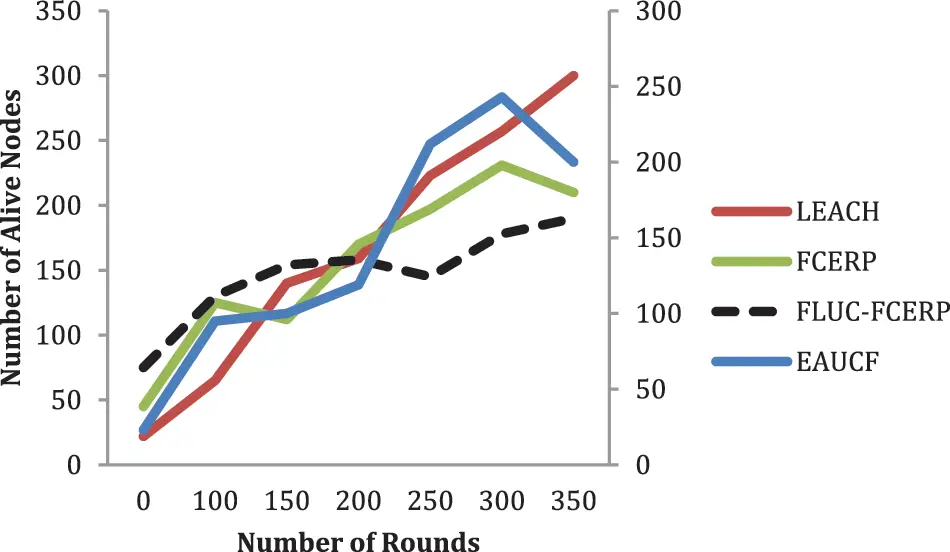Figure 7: Number of alive nodes

The lifetime of the network characteristics were studied by considering a number of diverse nodes as well as the position of BS in the centre of the workplace environment to properly evaluate FLUP-FCERP to certain other approaches. Also, the findings of this research are displayed in Table 2.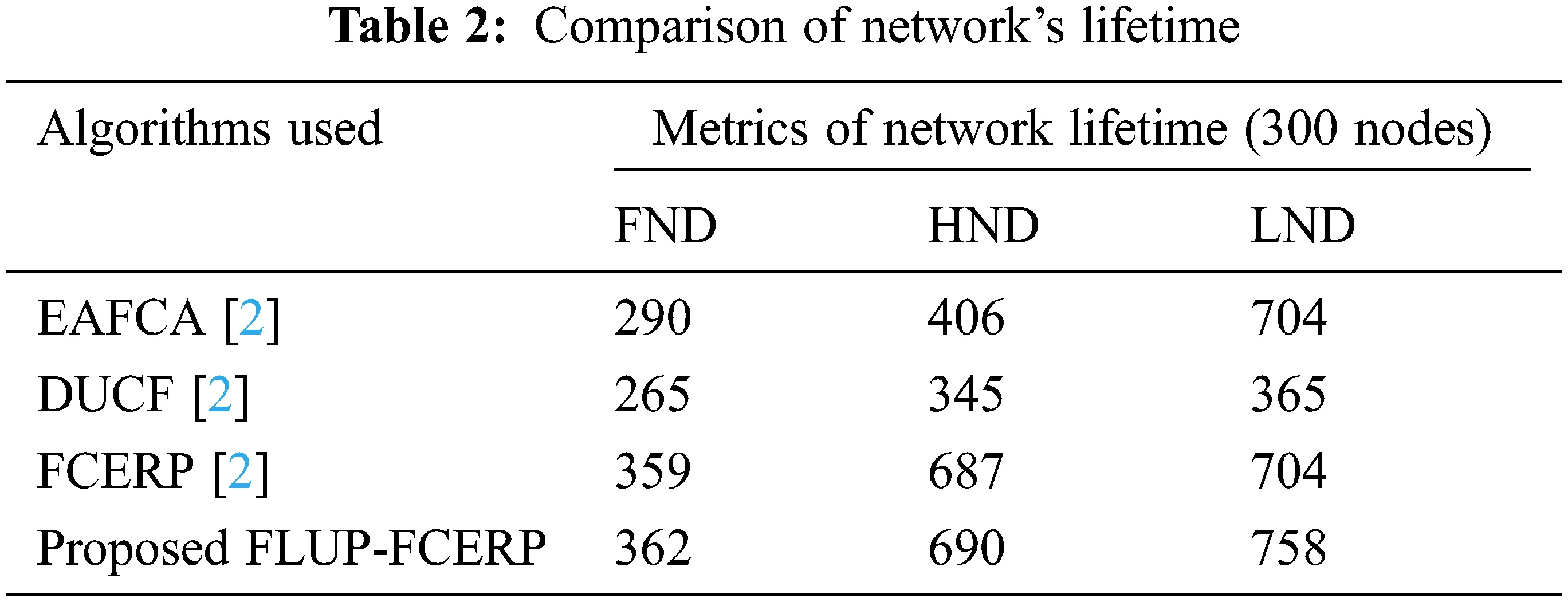In comparison with other types, FLIP-FCERP seems to have a better performance in terms of enhancing the lifetime of the network, as per the findings.

5  Conclusion

The proposed work suggested a fuzzy logic-based method for reducing the network energy consumption. Equally, massive fixed systems of network nodes were among the assertions considered. In order to test the hypothesis, a new method was implemented in 3 stages. For minimizing the energy consumption, the first step that was carried out was to use multi-hop communications. That particular stage was followed by an extraction stage in which the power hole problem was handled by an unequal clustering by shrinking the size of the cluster around BS and using fuzzy logic protocols to determine the competing radius. Fuzzy logic was used in the final phase. When estimating the distance to BS, CHs were chosen based on the nodes of remaining energy and concentrations. Fuzzy Logic-based clustering protocol FLUC (unequally clustered) and the Fuzzy Based Clustering and Energy-Efficient Routing Protocol (FCERP) algorithms were suggested. The algorithm was used to evaluate the scalability in terms of node count, node density, and BS location. The suggested FLUC-FCERP method decreased the amount of control packets broadcast, optimized FND, HND, and LND variables, and saved energy based on the assessments. By using the maximum power of CH node in combination with a set threshold limit had improved the performance of the system. Major limitation of the study was that the energy efficiency was achieved based on the best threshold value. In future, the real time node processing with deep learning algorithms can be tested to improve the power factors.

Funding Statement: The authors received no specific funding for this study.

Conflicts of Interest: The authors declare that they have no conflicts of interest to report regarding the present study.

## References

1. M. Adnan, L. Yang, T. Ahmad and Y. Tao, “An unequally clustered multihop routing protocol based on fuzzy logic for wireless sensor networks,” IEEE Access, vol. 9, pp. 38531–38545, 202
2. A. Zainab, M. Siddique and M. Waleed Ashour, “FCERP: A novel WSNs fuzzy clustering and energy efficient routing protocol,” Annals of Emerging Technologies in Computing (AETiC), vol. 6, no. 1, pp. 1–12, 202
3. S. Alpersert, A. Alchihabi and A. Yazici, “A two tier distributed fuzzy logic based protocol for efficient data aggregation in multihop wireless sensor networks,” IEEE Transactions on Fuzzy Systems, vol. 26, pp. 3615–3629, 2018.
4. A. Jain and A. Pathak, “Ant colony optimization and excess energy calculations based fast converging energy efficient routing algorithm for WSNs,” Wireless Personal Communication, vol. 109, no. 4, pp. 2305–2328, 2019.
5. T. Kaur and D. Kumar, “MACO-QCR: Multi objective ACO-based QoS-aware cross layer routing protocols in WSN,” IEEE Sensors Journal, vol. 21, no. 5, pp. 6775–6783, 2020.
6. X. Huan, K. S. Kim, S. Lee, E. G. Lim and A. Marshall, “A beaconless asymmetric energy efficient time synchronization scheme for resource-constrained multihop wireless sensor networks,” IEEE Transactions on Communications, vol. 68, no. 3, pp. 1716–1730, 2019.
7. S. Chamanian, S. Baghaee, H. Uluşan, O. Zorlu, E. Biyikoglu et al., “Implementation of energy-neutral operation on vibration energy harvesting WSN,” IEEE Sensors Journal, vol. 19, no. 8, pp. 3092–3099, 2019.
8. R. Ashween, B. Ramakrishnan and M. Milton Joe, “Energy efficient data gathering technique based on optimal mobile sink node selection for improved network life time in wireless sensor network (WSN),” Wireless Personal Communications, vol. 113, no. 4, pp. 2107–2126, 2020.
9. S. Q. Zhang, Y. Tao and J. J. Dai, “Multi-hop clustering routing protocol for energy harvesting wireless sensor networks,” Computer Engineering and Design, vol. 40, no. 3, pp. 611–616, 201
10. L. Zhang, Y. Lu, L. Chen and D. Dong, “Game theoretical algorithm for coverage optimization in wireless sensor networks,” in Proc. of the World Congress on Engineering, USA, pp. 1–4, 2008.
11. M. Huang, A. Liu, M. Zhao and T. Wang, “Multi working sets alternate covering scheme for continuous partial coverage in WSNs,” Peer-to-Peer Networking and Applications, vol. 12, no. 3, pp. 553–567, 2019.
12. S. Navrati, A. Roy and J. Shin, “Dynamic duty cycle and adaptive contention window based QoS-MAC protocol for wireless multimedia sensor networks,” Computer Networks, vol. 52, no. 13, pp. 2532–2542, 2008.
13. X. Huan, K. S. Kim, S. Lee, E. G. Lim and A. Marshall, “A beaconless asymmetric energy-efficient time synchronization scheme for resource constrained multi-hop wireless sensor networks,” IEEE Transactions on Communications, vol. 68, no. 3, pp. 1716–1730, 2019.
14. N. G. Palan, B. V. Barbadekar and P. Suahs, “Low energy adaptive clustering hierarchy (LEACH) protocol: A retrospective analysis,” in Proc. Int. Conf. on Inventive Systems and Control (ICISC), India, pp. 1–12, 2017.
15. H. Mostafaei, “Energy efficient algorithm for reliable routing of wireless sensor networks,” IEEE Transactions on Industrial Electronics, vol. 66, no. 7, pp. 5567–5575, 2019.
16. H. Mostafaei and M. S. Obaidat, “Learning automaton based self protection algorithm for wireless sensor networks,” IET Networks, vol. 7, no. 5, pp. 353–361, 2018.
17. H. E. Alami and A. Najid, “ECH: An enhanced clustering hierarchy approach to maximize lifetime of wireless sensor networks,” IEEE Access, vol. 7, pp. 107142–107153, 2019.
18. H. E. Alami and A. Najid, “Mrouting routing technique to minimize energy consumption and packet loss in WSNs with mobile sink,” IET Network, vol. 7, no. 6, pp. 422–428, 20
19. S. A. Sert and A. Yazici, “Optimizing the performance of rule based fuzzy routing algorithms in wireless sensor networks, in Proc. IEEE Int. Conf. Fuzzy System, New Orleans, USA, pp. 1–6, 20
20. S. A. Sert, A. Alchihabi and A. Yazici, “A two-tier distributed fuzzy logic based protocol for efficient data aggregation in multihop wireless sensor networks,” IEEE Transactions on Fuzzy Systems, vol. 26, no. 6, pp. 3615–3629, 2018.
21. G. Vijayalakshmi, M. A. Bennet, P. Shenbagavalli, M. Vijayalakshmi and S. Saranya, “Cluster head election mechanism-based on fuzzy logic (CHEF) with TDMA in WSN,” International Journal on Smart Sensing and Intelligent Systems, vol. 10, no. 1, pp. 395–413, 2017.
22. A. Hamzah, M. Shurman, O. Al-Jarrah and E. Taqieddin, “Energy efficient fuzzy logic based clustering technique for hierarchical routing protocols in wireless sensor networks,” Sensors, vol. 19, pp. 561, 2019.
23. B. Balakrishnan and S. Balachandran, “FLECH: Fuzzy logic based energy efficient clusteringhierarchy for nonuniform wireless sensor networks,” Wireless Communications and Mobile Computing, vol. 2017, 2017.
24. J. Wang, X. J. Gu, W. Liu, A. K. Sangaiah and H. J. Kim, “An empower hamilton loop based data collection algorithm with mobile agent for WSNs,” Human-Centric Computing and Information Sciences, vol. 9, no. 1, pp. 1–14, 2019.
25. J. Wang, Y. Gao, C. Zhou, S. Sherratt and L. Wang, “Optimal coverage multi-path scheduling scheme with multiple mobile sinks for WSNs,” Computers, Materials & Continua, vol. 62, no. 2, pp. 695–711, 2020.

J. Gobinath, S. Hemajothi and J. S. Leena Jasmine, "Energy-efficient routing protocol with multi-hop fuzzy logic for wireless networks," Intelligent Automation & Soft Computing, vol. 36, no.2, pp. 2457–2471, 2023.This work is licensed under a Creative Commons Attribution 4.0 International License , which permits unrestricted use, distribution, and reproduction in any medium, provided the original work is properly cited.

View

Like

## Related articles

• Martínez Corral L, E Martínez-Rubin,...
• R. Abdeljabar
• Cheng Zhu, Rodger P. McEver